Next: Implementation Up: Krawczyk method for solving Previous: Krawczyk method for solving   Contents

Mathematical background

Let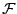be a system ofequations in theunknowns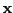. Let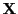be a range vector forand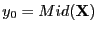. Let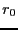be the norm of the matrix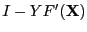. Let the following iterative scheme for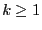: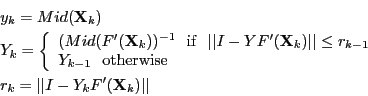Let define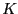as: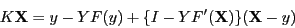If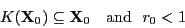then the previous iterative scheme will converge to the unique solution of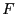in. The procedure described in section 3.1.1 enable to verify if the scheme will be convergent.

Jean-Pierre Merlet 2012-12-20